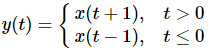Courses

Solved Examples - Basic System Properties Notes | Study Digital Signal Processing - Electrical Engineering (EE)

Electrical Engineering (EE): Solved Examples - Basic System Properties Notes | Study Digital Signal Processing - Electrical Engineering (EE)

The document Solved Examples - Basic System Properties Notes | Study Digital Signal Processing - Electrical Engineering (EE) is a part of the Electrical Engineering (EE) Course Digital Signal Processing.
All you need of Electrical Engineering (EE) at this link: Electrical Engineering (EE)

Example 1 − Check whether y(t) = x∗(t) is linear or non-linear.

Solution − The function represents the conjugate of input. It can be verified by either first law of homogeneity and law of additivity or by the two rules. However, verifying through rules is lot easier, so we will go by that.

If the input to the system is zero, the output also tends to zero. Therefore, our first condition is satisfied. There is no non-linear operator used either at the input nor the output. Therefore, the system is Linear.

Example 2 − Check whetheris linear or non linear

Solution − Clearly, we can see that when time becomes less than or equal to zero the input becomes zero. So, we can say that at zero input the output is also zero and our first condition is satisfied.

Again, there is no non-linear operator used at the input nor at the output. Therefore, the system is Linear.

Example 3 − Check whether y(t) = sint.x(t) is stable or not.

Solution − Suppose, we have taken the value of x(t) as 3. Here, sine function has been multiplied with it and maximum and minimum value of sine function varies between -1 to +1.

Therefore, the maximum and minimum value of the whole function will also vary between -3 and +3. Thus, the system is stable because here we are getting a bounded input for a bounded output.

The document Solved Examples - Basic System Properties Notes | Study Digital Signal Processing - Electrical Engineering (EE) is a part of the Electrical Engineering (EE) Course Digital Signal Processing.
All you need of Electrical Engineering (EE) at this link: Electrical Engineering (EE)Use Code STAYHOME200 and get INR 200 additional OFF Use Coupon Code

Top Courses for Electrical Engineering (EE)Digital Signal Processing

3 videos|50 docs|54 tests

Top Courses for Electrical Engineering (EE)Track your progress, build streaks, highlight & save important lessons and more!

,

,

,

,

,

,

,

,

,

,

,

,

,

,

,

,

,

,

,

,

,

;